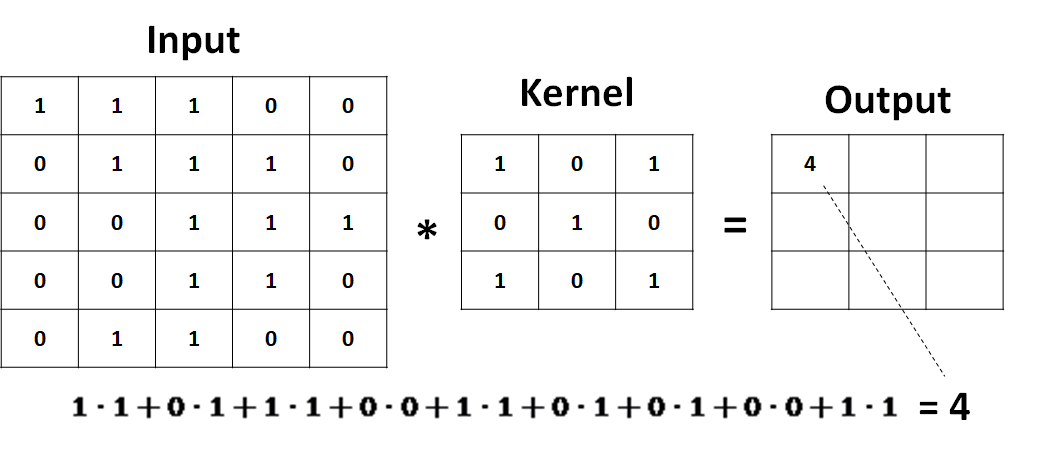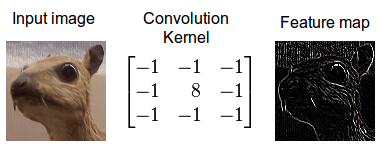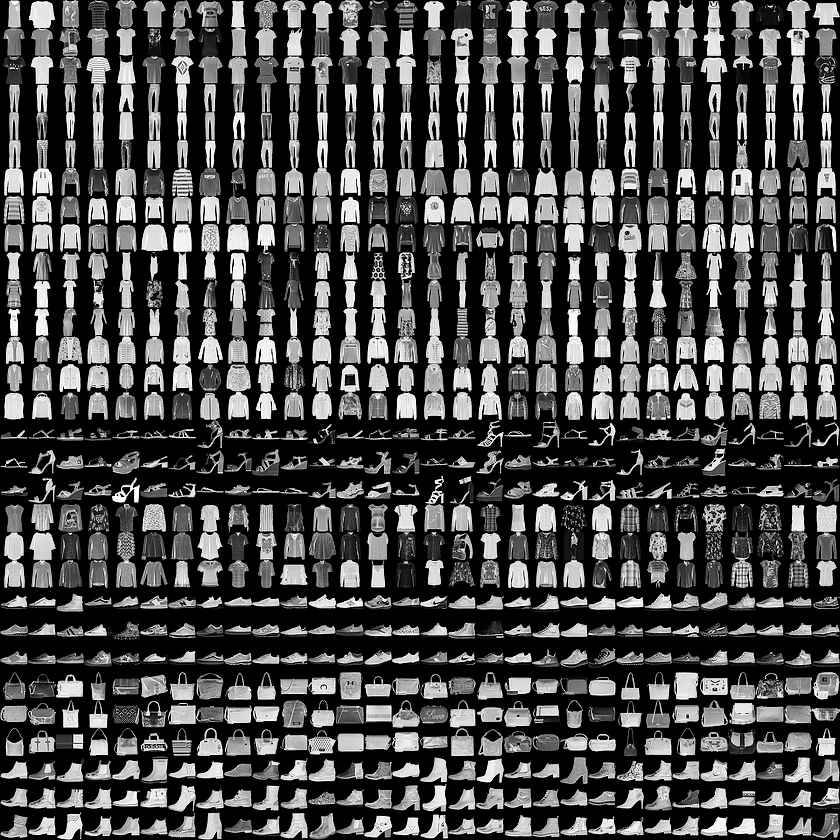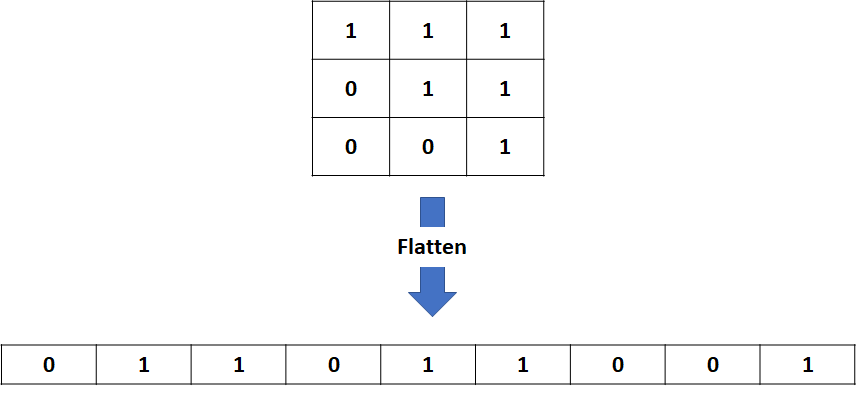# Deep Learning with Keras – Part 5: Convolutional Neural Networks

0
7065Image by Ahmed Gad from Pixabay

## Motivation

In the previous articles, we solved problems with numeric and categorical data, and we learned the different transformations needed for each. Regarding images, we investigated a simple hack that resizes each image into an array of pixels and feed it to the input layer. The approach worked well and we reached around 97% accuracy on MNIST dataset.

However, dealing with large images with more complex patterns is completely different. Scientists struggled to reach an acceptable performance to even classify a dog vs cat image. Images like that contain many features that are related in a specific way. For example: some set of pixels in a given order define an edge, a circle, a nose, a mouth, etc. Therefore, we need a special kind of layer that detects these relations.

Here comes the role of the convolution layer. It is a neural network layer that scans an image, and extracts a set of features from it. Normally, we would accumulate those layers to learn more complex features. This way, the first layers learn very basic features such as horizontal edges, vertical edges, lines, etc. The deeper we go the more complex become the features. Layers will then be able to combine low level features into high level ones. For example: edges and curves could be combined to detect shapes of different heads, noses, ears, etc.

Convolution layers made a really high impact on the whole machine and deep learning fields. It allowed us to automated very complex tasks with human-level performance or even outperform humans in some cases. So, pay close attention you are going to have a very powerful weapon in your arsenal.

## Image Kernels

An kernel (or filter) is simply a small matrix applied to an image with the convolution operator.

The process is as follows:

1. a small matrix of shape (k1, k2) slides over the input,
2. applies a pairwise multiplication on the two matrices,
3. the sum of the resulting matrix is taken and the result is put into the final matrix output

See the image for better clarification:The convolution operator

Applying a filter to an image extracts some features from it. The following image shows how a simple kernel detects edges.Image kernels

The question here is how to get those numbers inside the kernel? Well, why don’t we make the neural network learn the best kernels to classify a set of images? This is core concept behind convolutional neural networks. Convolutional layers act as automatic feature extractors that are learned from the data.

## Problem Definition

In this article we will train a convolutional neural network to classify clothes types from the fashion MNIST dataset.

Fashion-MNIST is a dataset of Zalando’s article images consisting of a training set of 60,000 examples and a test set of 10,000 examples. Each example is a 28×28 grayscale image, associated with a label from 10 classes.Image Source: https://github.com/zalandoresearch/fashion-mnist

The labels are:Output Labels

## Loading the Data

Again we will use Keras to download our data.

``````from keras.datasets import fashion_mnist
(X_train, y_train), (X_test, y_test) = fashion_mnist.load_data()``````

## Preprocessing Data

We need to do three simple modifications to our data:

1. Transform the y_train and y_test into one hot encoded versions
2. Reshape our images into (width, height, number of channels). Since we are dealing with gray scale images the number of channels will be one
3. Scale our images by dividing with 255
``````# to categorical
from keras.utils import to_categorical
y_train_final = to_categorical(y_train)
y_test_final = to_categorical(y_test)

# reshape
X_train_final = X_train.reshape(-1, 28, 28, 1) / 255.
X_test_final = X_test.reshape(-1, 28, 28, 1) / 255.``````

## Building the Network

Building a convolutional neural network is not different that building a normal one. The one difference here is that we do not need to reshape our images, because convolutional layers work with 2D images.

``````from keras import models, layers

model = models.Sequential()
model.add(layers.Conv2D(8, (3, 3), activation='relu', input_shape=(28, 28, 1)))
model.add(layers.Flatten())
model.add(layers.Dense(128, activation='relu'))
model.add(layers.Dense(10, activation='softmax'))

model.compile('rmsprop', 'categorical_crossentropy', metrics=['acc'])``````

The only new thing here is the first layer and the Flattern layer. We use a Conv2D that is the 2D convolution layer for 2D images. The parameters are the following:

1. The number of kernels/filters to learn. Here we used 32 kernels. Imagine that each one of these kernels will learn a simple feature like vertical edge detection, horizontal edge detection, etc
2. The size of the kernel. Here we used a 3 by 3 matrix.
3. The activation function applied to the final output
4. The input shape where 28 is the image width and height and 1 is the number of channels (1 since it is a gray scale image, for RGB we use 3)

Since the output of a convolution is a multidimensional matrix, we need to reshape the output (as we did before with a regular neural network). The flatten layer here does the same, it unfolds the matrix into an array that is then fed to the next layer.Flatten Layers

Note: We used a softmax output layer of 10 Dense connected neurons since we have 10 labels to learn.

## Training the Network

As before, we just have to call the fit method:

``history = model.fit(X_train_final, y_train_final, validation_split=0.2, epochs=3)``
` Train on 48000 samples, validate on 12000 samples Epoch 1/3 48000/48000 [==============================] - 19s 395us/step - loss: 0.4352 - acc: 0.8480 - val_loss: 0.3410 - val_acc: 0.8805 Epoch 2/3 48000/48000 [==============================] - 16s 332us/step - loss: 0.3132 - acc: 0.8909 - val_loss: 0.3213 - val_acc: 0.8873 Epoch 3/3 48000/48000 [==============================] - 17s 362us/step - loss: 0.2845 - acc: 0.9016 - val_loss: 0.3122 - val_acc: 0.8931 `

With a very simple convolutional network we were able to reach 90% accuracy. The network could be improved for sure by adding more advanced layers and maybe some regularization techniques, but we will keep this for later articles.

## Challenge

Try training a simple neural network (do not use convolutions) on the same dataset. Report your results in the comments section below.

## Final Thoughts

In this article we learned the very basics of convolutional neural networks. We learned that they are used to automatically extract image features to yield higher accuracy than the standard fully connected networks.

Note: This is a guest post, and opinion in this article is of the guest writer. If you have any issues with any of the articles posted at www.marktechpost.com please contact at [email protected]

This site uses Akismet to reduce spam. Learn how your comment data is processed.